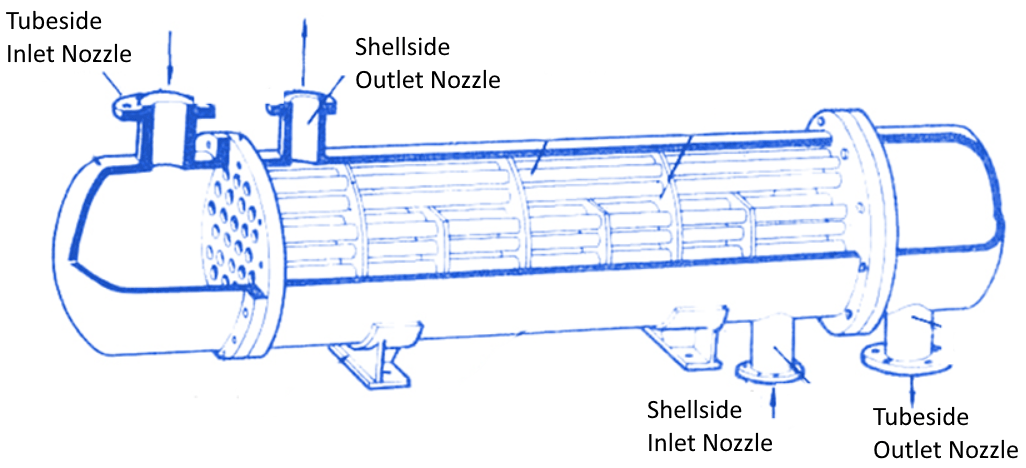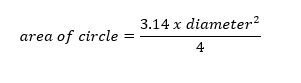# How Are Nozzles Sized, And When Do We Need Impingement Protection?## How Are Nozzles Sized, And When Do We Need Impingement Protection?

You may have wondered how nozzles are sized for heat exchangers – why are some 12 inches in diameter while others are 4, 6, or 24 inches?  Well, it mostly comes down the how much flow is going through them.  Higher flows need larger diameter nozzles – not unlike a four-lane highway vs a side-street.

In general, our customers specify the nozzle size they need to match their piping, but we also need to check this sizing during our thermal design to ensure nozzles are big enough.If the nozzle size is too small, the fluid velocities inside the nozzles can become very large and will cause erosion at the nozzles, nozzle interface to shell cylinder, and to the tubes themselves.  In the picture to the right showing a narrowed pipe, the flowrate is the same from left to right throughout, but you intuitively know that the velocity in the middle `pinched’ section will be much higher.

Undersized nozzles also cause more pressure-loss through them, which costs energy in terms of more pumping power required, but also less opportunity for us to optimize the heat exchanger design for heat transfer.

Choosing nozzle sizes by the amount of flow is not accurate enough – there are too many different types of fluids in both liquid and vapour states, so we use a formula known as  (pronounced rho-vee-squared).

What this formula means is we take the process density (rho) and multiple it by the velocity (v) twice. We would already know the density because the customer provides us that along with the flowrate, but do need to calculate the velocity ourselves (next page).

Fluid velocity inside a nozzle is calculated from the flowrate divided by the cross-sectional flow area of the nozzle:…but we don’t have the area yet you say?  Well, to get the nozzle (flow) area, you may remember this one from grade school:Alright, now we can do some math. I will assume nozzle size is 12 (ignoring units):

Area = 3.14 x 12 x 12 divided by 4
Area = 113

Now we can jump back to the velocity equation, and assuming flowrate is 500 (ignoring units again):

Velocity = 500 divided by 113
Velocity = 4.4

However, if we use a smaller nozzle size like 4, instead of 12:

Area = 3.14 x 4 x 4 / 4
Area = 13

Velocity = 500 divided by 13
Velocity = 38.

Hopefully, everyone is still with me here – we are heading into the final stretch.  Now, using water with a density (rho) of 62, the rho-vee-squared values can be calculated for both the 12 and 4 inch nozzles.

12 inch nozzle, rho-vee-squared = 62 x 4.4 x 4.4 = 1,200

4 inch nozzle, rho-vee-squared = 62 x 38 x 38 = 89,528

The rho-vee-squared numbers above are essentially showing how energetic the flow is through the nozzles, and you can see that the smaller 4 inch nozzle has a value about 75x higher than the 12 inch nozzle.

So how do we know if a rho-vee-squared number is safe? We reference the TEMA (Tubular Exchanger Manufacturers Association) handbook (section RCB-4.6) for suggested values:### Our location

Lorem ipsum dolor sit amet, consectetur elit. Quisque quis quam ipsum